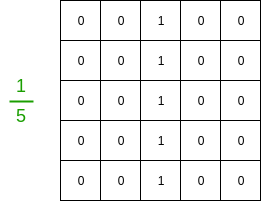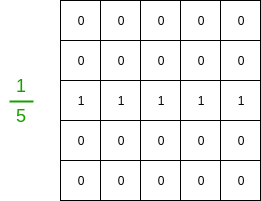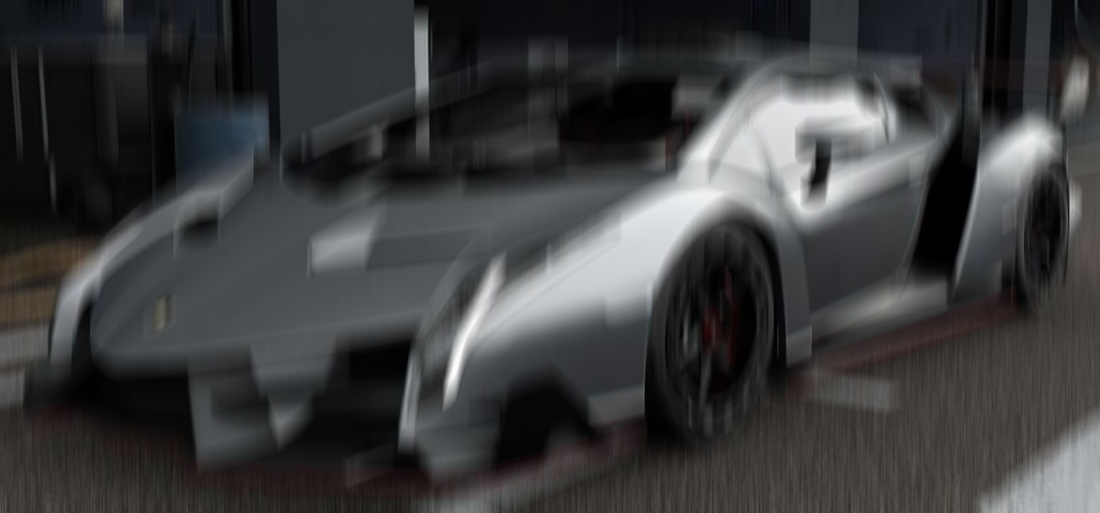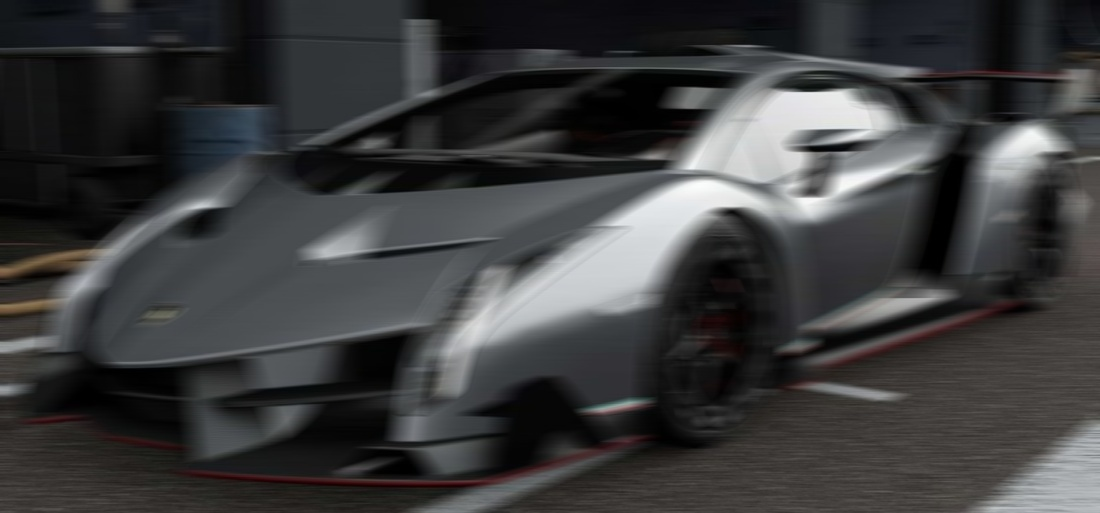GeeksforGeeks App
Open AppBrowser
Continue

# OpenCV | Motion Blur in Python

This article explains how to add blur to an image using OpenCV. Motion blur is a specific type of blur used to lend a directed blur effect to images.

The Motion Blur Filter
Applying motion blur to an image boils down to convolving a filter across the image. Sample 5*5 filter filters are given below.

Vertical:Horizontal:The greater the size of the filter, the greater will be the motion blur effect. Further, the direction of 1’s across the filter grid is the direction of the desired motion. To customize a motion blur in a specific vector direction, e.g. diagonally, simply place the 1’s along the vector to create the filter.

Code
Consider the following image of a car.Code: Python code for applying a motion blur effect on the image.

 `# loading library``import` `cv2``import` `numpy as np`` ` `img ``=` `cv2.imread(``'car.jpg'``)`` ` `# Specify the kernel size.``# The greater the size, the more the motion.``kernel_size ``=` `30`` ` `# Create the vertical kernel.``kernel_v ``=` `np.zeros((kernel_size, kernel_size))`` ` `# Create a copy of the same for creating the horizontal kernel.``kernel_h ``=` `np.copy(kernel_v)`` ` `# Fill the middle row with ones.``kernel_v[:, ``int``((kernel_size ``-` `1``)``/``2``)] ``=` `np.ones(kernel_size)``kernel_h[``int``((kernel_size ``-` `1``)``/``2``), :] ``=` `np.ones(kernel_size)`` ` `# Normalize.``kernel_v ``/``=` `kernel_size``kernel_h ``/``=` `kernel_size`` ` `# Apply the vertical kernel.``vertical_mb ``=` `cv2.filter2D(img, ``-``1``, kernel_v)`` ` `# Apply the horizontal kernel.``horizonal_mb ``=` `cv2.filter2D(img, ``-``1``, kernel_h)`` ` `# Save the outputs.``cv2.imwrite(``'car_vertical.jpg'``, vertical_mb)``cv2.imwrite(``'car_horizontal.jpg'``, horizonal_mb)`

Output

Vertical Blur:Horizontal Blur:My Personal Notes arrow_drop_up